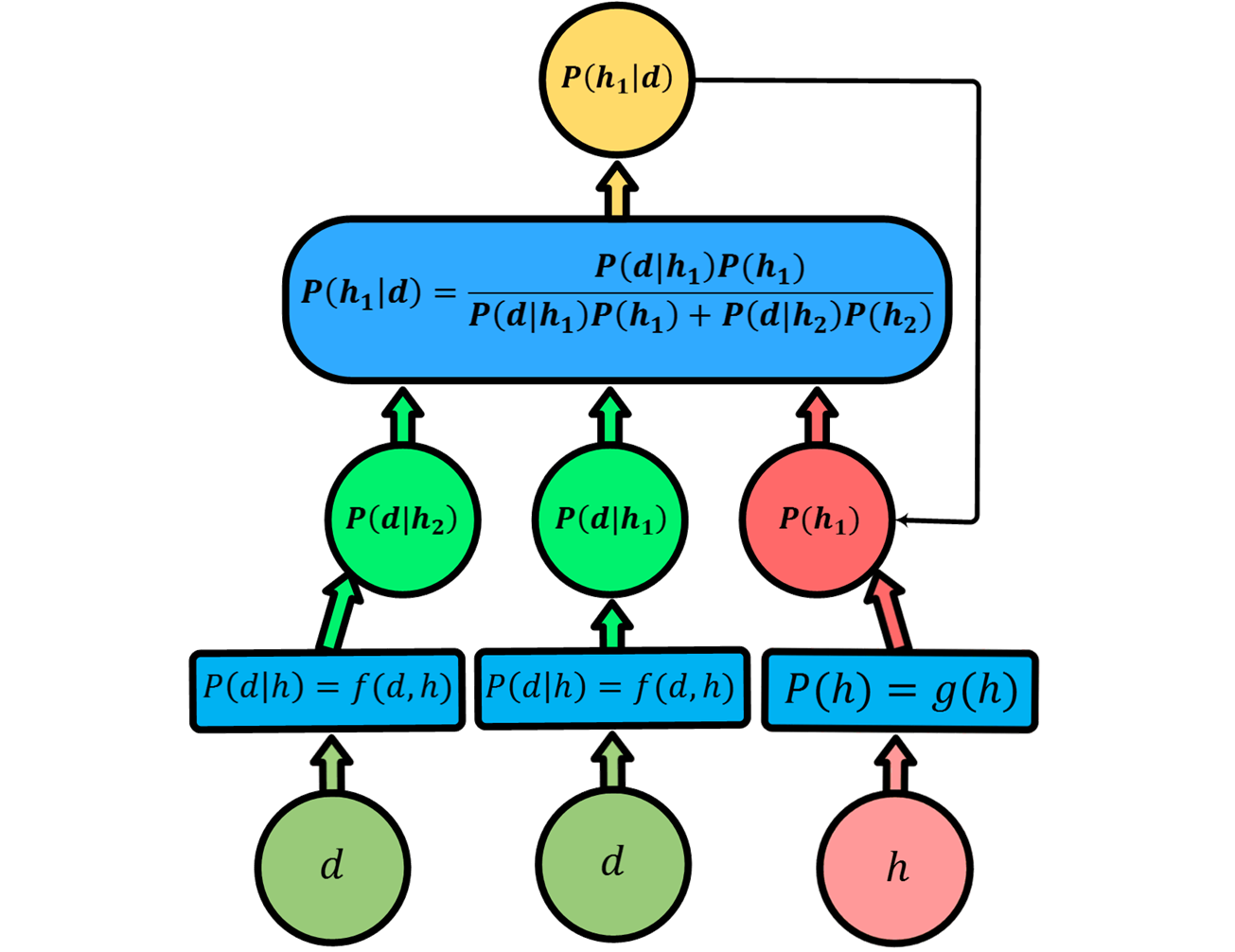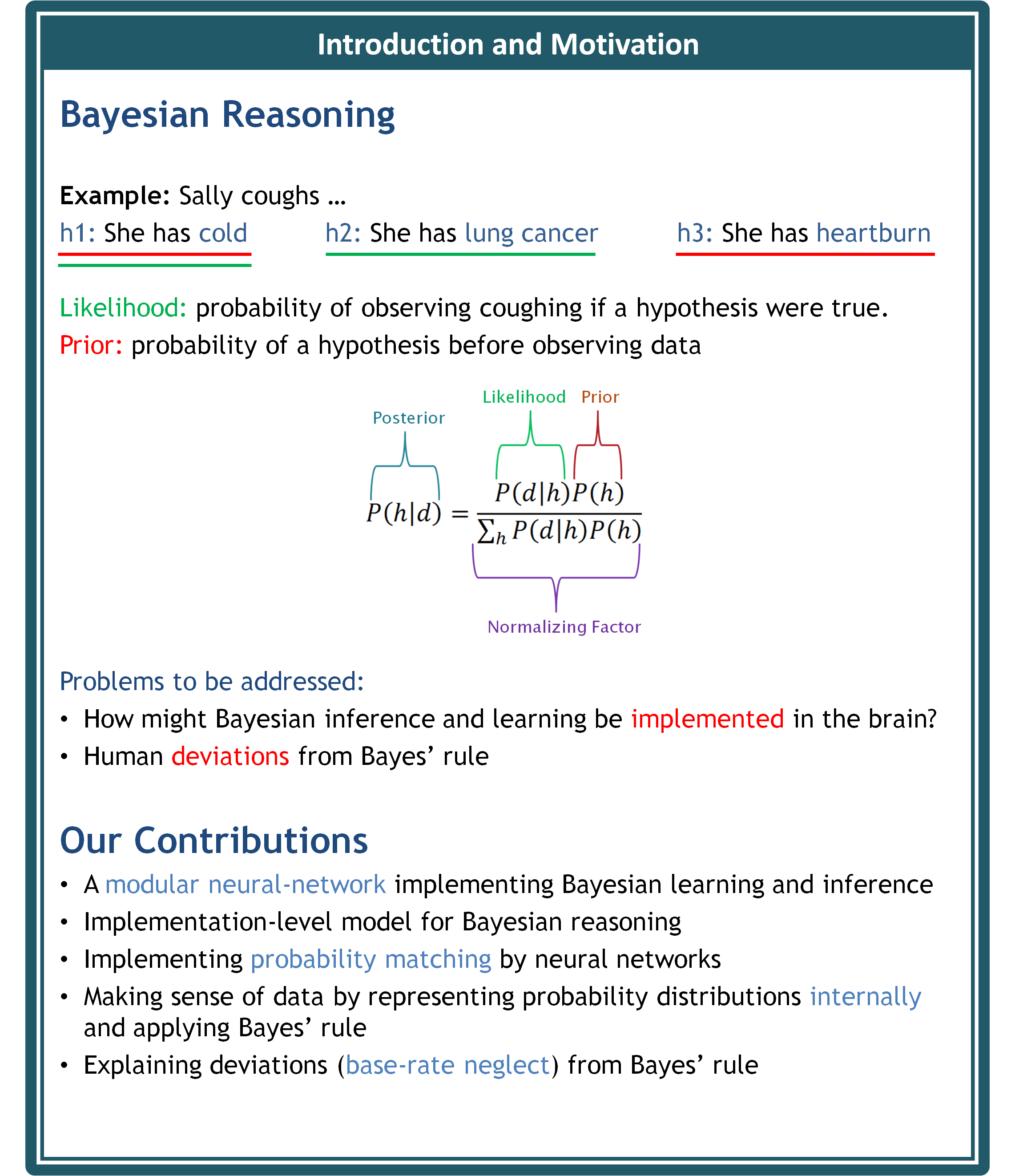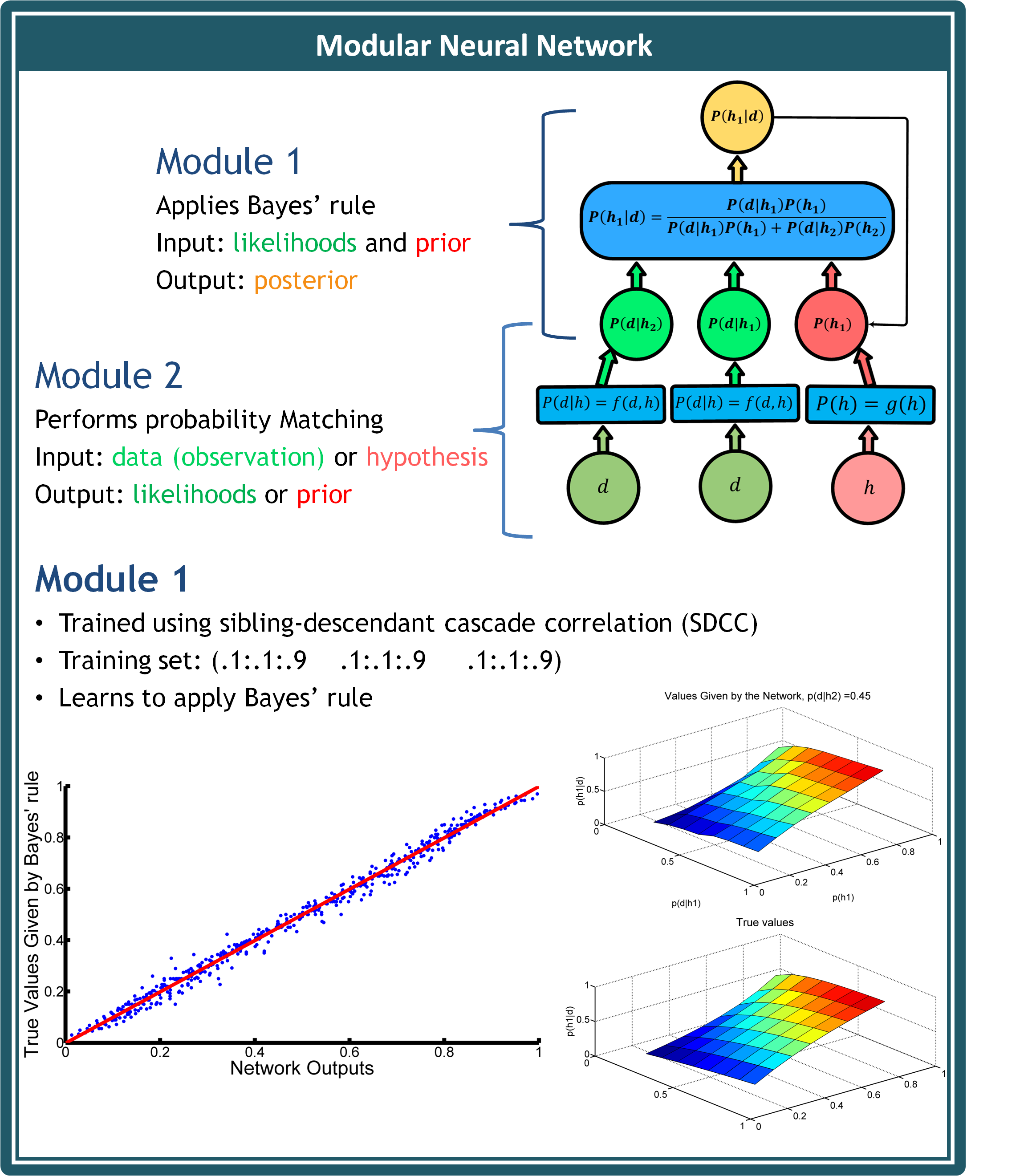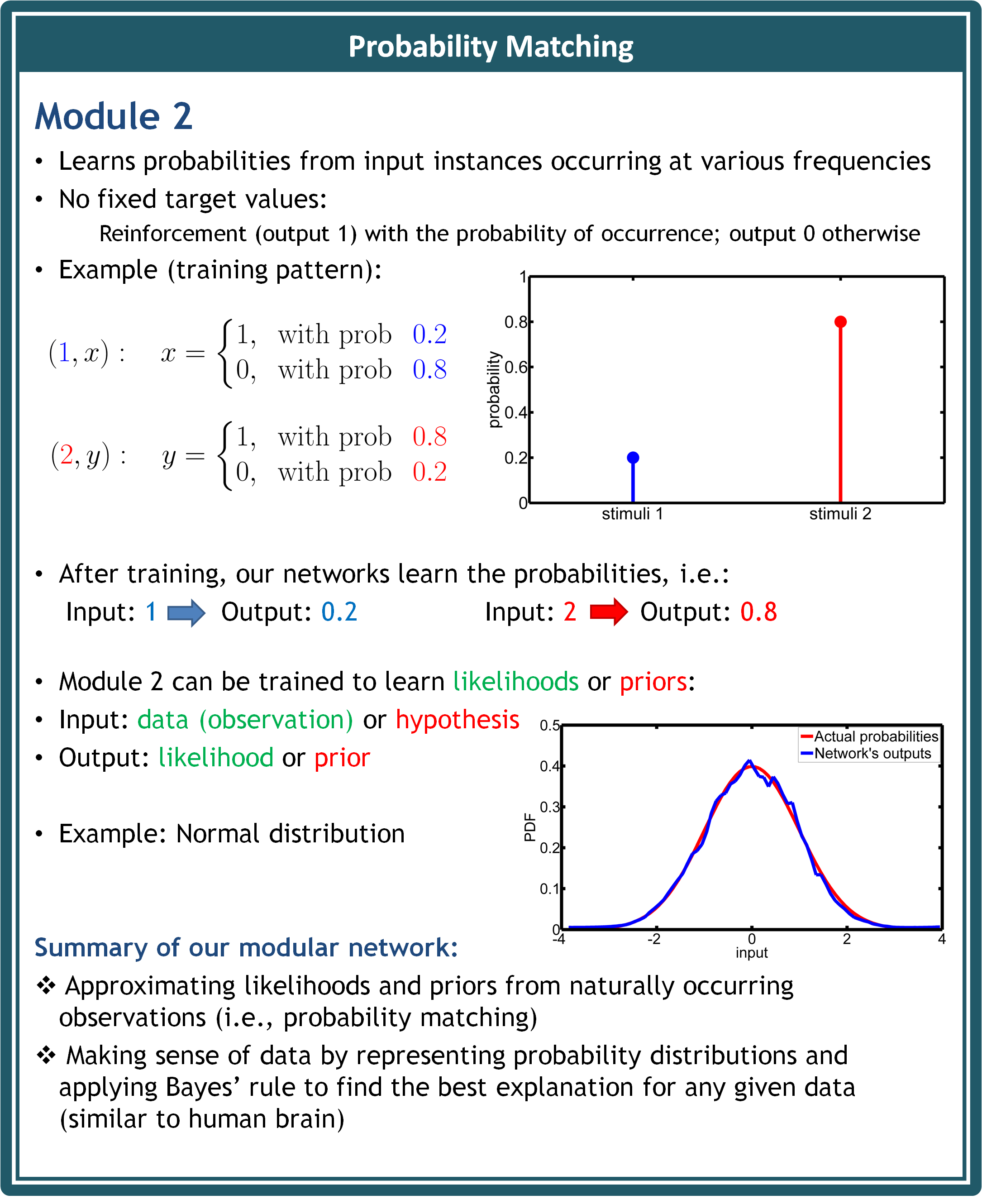## Neural Implementation of Bayesian Learning and Inference

 Bayesian models of cognition hypothesize that human brains make sense of data by innately representing probability distributions and applying Bayes' rule to find the best explanation for any given data. Understanding the neural mechanisms underlying the probabilistic models is of particular importance because these models offer only a computational framework. We propose a constructive neural-network model which estimates and represents probability distributions from observable inputs. We use a form of operant learning, where the underlying probabilities are learned from positive and negative reinforcements of the inputs. Our model is psychologically plausible because similar to humans, it innately learns to represent probabilities without receiving any direct information about them from the external world. Moreover, we show that our neural implementation of probability matching can be paired with a neural module applying Bayes' rule in order to form a complete neural scheme which can account for human Bayesian learning and inference. We also provide a novel explanation of base-rate neglect, the most well-documented deviation from Bayes' rule, by modelling it as a weight decay mechanism which increases entropy. For more details, please choose the appropriate tab above.35th Annual Conference of the Cognitive Science Society, Berlin, Germany, Cognitive Science Society, pp. 2686-2691, Aug 2013. RTF Tagged XML BibTex Google Scholar